# Check Machin-like formulae with arbitrary-precision arithmetic

January 3, 2019
By

Want to share your content on R-bloggers? click here if you have a blog, or here if you don't.

Happy New Year to all of you! Let us start the year with something for your inner maths nerdFor those of you who don’t yet know Rosetta Code: it is a real cool site where you can find lots of interesting code examples in all kinds of different languages for many different tasks. Of course R is also present big time (at the time of writing 426 code examples!): Rosetta Code for R.

The name of the site is inspired by the famous Rosetta Stone of Ancient Egypt which is inscribed with three different versions of the same text: in Ancient Egyptian hieroglyphs, Demotic script, and Ancient Greek script which proved invaluable in deciphering Egyptian hieroglyphs and thereby opening the window into ancient Egyptian history.

Now, a few days a ago I again added an example (for the other tasks I solved I will write more posts in the future, so stay tuned!). The task is to verify the correctness of Machin-like formulae using exact arithmetic.

A little bit of mathematical background is in order, so Wikipedia to the rescue:

Machin-like formulae are a popular technique for computingto a large number of digits. They are generalizations of John Machin]s formula from 1706: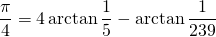which he used to computeto 100 decimal places.

Machin-like formulae have the formwhere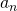andare positive integers such that,is a signed non-zero integer, andis a positive integer.

The exact task is to verify that the following Machin-like formulae are correct by calculating the value of tan (right hand side) for each equation using exact arithmetic and showing they equal one: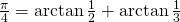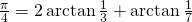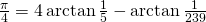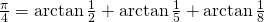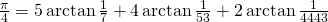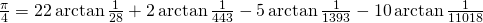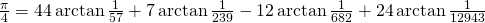The same should be done for the last and most complicated case…… but it should be confirmed that the following, slightly changed, formula is incorrect by showing tan (right hand side) is not one:This is what I contributed to Rosetta Code:

library(Rmpfr)
prec <- 1000 # precision in bits
%:% <- function(e1, e2) '/'(mpfr(e1, prec), mpfr(e2, prec)) # operator %:% for high precision division
# function for checking identity of tan of expression and 1, making use of high precision division operator %:%
tanident_1 <- function(x) identical(round(tan(eval(parse(text = gsub("/", "%:%", deparse(substitute(x)))))), (prec/10)), mpfr(1, prec))

tanident_1( 1*atan(1/2)    +  1*atan(1/3) )
##  TRUE
tanident_1( 2*atan(1/3)    +  1*atan(1/7))
##  TRUE
tanident_1( 4*atan(1/5)    + -1*atan(1/239))
##  TRUE
tanident_1( 5*atan(1/7)    +  2*atan(3/79))
##  TRUE
tanident_1( 5*atan(29/278) +  7*atan(3/79))
##  TRUE
tanident_1( 1*atan(1/2)    +  1*atan(1/5)   +   1*atan(1/8) )
##  TRUE
tanident_1( 4*atan(1/5)    + -1*atan(1/70)  +   1*atan(1/99) )
##  TRUE
tanident_1( 5*atan(1/7)    +  4*atan(1/53)  +   2*atan(1/4443))
##  TRUE
tanident_1( 6*atan(1/8)    +  2*atan(1/57)  +   1*atan(1/239))
##  TRUE
tanident_1( 8*atan(1/10)   + -1*atan(1/239) +  -4*atan(1/515))
##  TRUE
tanident_1(12*atan(1/18)   +  8*atan(1/57)  +  -5*atan(1/239))
##  TRUE
tanident_1(16*atan(1/21)   +  3*atan(1/239) +   4*atan(3/1042))
##  TRUE
tanident_1(22*atan(1/28)   +  2*atan(1/443) +  -5*atan(1/1393) + -10*atan(1/11018))
##  TRUE
tanident_1(22*atan(1/38)   + 17*atan(7/601) +  10*atan(7/8149))
##  TRUE
tanident_1(44*atan(1/57)   +  7*atan(1/239) + -12*atan(1/682)  +  24*atan(1/12943))
##  TRUE

tanident_1(88*atan(1/172)  + 51*atan(1/239) +  32*atan(1/682)  +  44*atan(1/5357) + 68*atan(1/12943))
##  TRUE
tanident_1(88*atan(1/172)  + 51*atan(1/239) +  32*atan(1/682)  +  44*atan(1/5357) + 68*atan(1/12944))
##  FALSE


As you can see all statements are TRUE except for the last one!

In the code I make use of the Rmpfr package (from Martin Maechler of ETH Zürich, Switzerland) which is based on the excellent GMP (GNU Multiple Precision) library. I define a new infix operator %:% for high-precision division and after that convert all standard divisions in the formulae to high-precision divisions and calculate the tan. Before I check if the result is identical to one I round it to 100 decimal places which is more than enough given the precision of, so about 300 decimal places, in the example.

Please let me know in the comments what you think of this approach and whether you see room for improvement for the code – Thank you!

R-bloggers.com offers daily e-mail updates about R news and tutorials about learning R and many other topics. Click here if you're looking to post or find an R/data-science job.
Want to share your content on R-bloggers? click here if you have a blog, or here if you don't.